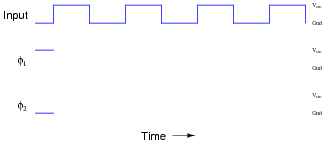# Switched Capacitor Circuitry

## Digital Circuits

• #### Question 1

Write an equation describing the precise mathematical relationship between electric charge (Q), capacitance (C), and voltage (V).

• #### Question 2

What would happen if a 10 μF capacitor, charged to a voltage of 6 volts, were connected to a completely discharged (0 volts) 22 μF capacitor?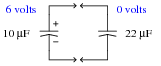What would the voltage(s) be across each capacitor, after enough time had passed for complete equalization to occur?

• #### Question 3

What would happen to the voltage across capacitor C2 if the following steps were followed, over and over again:Connect capacitor C1 to battery, allow to fully charge
Disconnect capacitor C1 from battery
Connect capacitor C1 to capacitor C2, allow for charges to equalize
Disconnect capacitor C1 from capacitor C2
Repeat
• #### Question 4

Describe what happens to Vout (the voltage across capacitor C4) as time goes on, assuming the relay is continuously toggled by the oscillator circuit at a high frequency. Assume that the input voltage (Vin) is constant over time: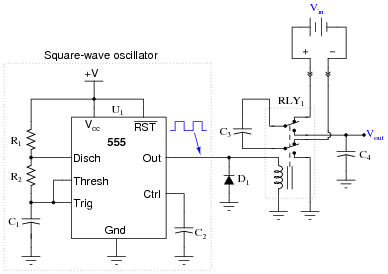This type of circuit is often referred to as a flying capacitor circuit, with C3 being the “flying” capacitor. Explain why this is, and what possible benefit might be realized by using a flying capacitor circuit to sample a voltage.

• #### Question 5

Suppose an engineer decided to use a flying capacitor circuit to sample voltage across a shunt resistor, to measure AC current from an electrical generator: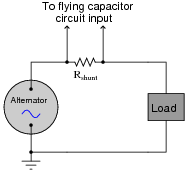The frequency of the alternator’s output is 50 Hz. How does this affect the design of the flying capacitor circuit, so we ensure a fairly accurate reproduction of the AC signal at the output of the flying capacitor circuit? Generalize your answer to cover all conditions where the input signal varies over time.

• #### Question 6

In this circuit, a capacitor is alternately connected to a voltage source, then a load, by means of two MOSFET transistors that are never conducting at the same time: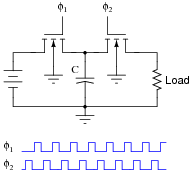Note: the φ1 and φ2 pulse signals are collectively referred to as a non-overlapping, two-phase clock.

Consider the average amount of current through the load resistor, as a function of clock frequency. Assume that the “on” resistance of each MOSFET is negligible, so that the time required for the capacitor to charge is also negligible. As the clock frequency is increased, does the load resistor receive more or less average current over a span of several clock cycles? Here is another way to think about it: as the clock frequency increases, does the load resistor dissipate more or less power?

Now suppose we have a simple two-resistor circuit, where a potentiometer (connected as a variable resistor) throttles electrical current to a load:It should be obvious in this circuit that the load current decreases as variable resistance R increases. What might not be so obvious is that the aforementioned switched capacitor circuit emulates the variable resistor R in the second circuit, so that there is a mathematical equivalence between f and C in the first circuit, and R in the second circuit, so far as average current is concerned. To put this in simpler terms, the switched capacitor network behaves sort of like a variable resistor.

Calculus is required to prove this mathematical equivalence, but only a qualitative understanding of the two circuits is necessary to choose the correct equivalency from the following equations. Which one properly describes the equivalence of the switched capacitor network in the first circuit to the variable resistor in the second circuit?

 R = f C R = C f R = 1 fC R = fC

Be sure to explain the reasoning behind your choice of equations.

• #### Question 7

In this circuit, a capacitor is alternately connected in series between a voltage source and a load, then shorted, by means of two MOSFET transistors that are never conducting at the same time: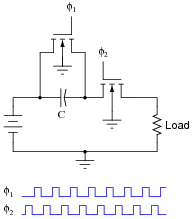Note: the φ1 and φ2 pulse signals are collectively referred to as a non-overlapping, two-phase clock.

Consider the average amount of current through the load resistor, as a function of clock frequency. Assume that the “on” resistance of each MOSFET is negligible, so that the time required for the capacitor to charge is also negligible. As the clock frequency is increased, does the load resistor receive more or less average current over a span of several clock cycles? Here is another way to think about it: as the clock frequency increases, does the load resistor dissipate more or less power?

Now suppose we have a simple two-resistor circuit, where a potentiometer (connected as a variable resistor) throttles electrical current to a load:It should be obvious in this circuit that the load current decreases as variable resistance R increases. What might not be so obvious is that the aforementioned switched capacitor circuit emulates the variable resistor R in the second circuit, so that there is a mathematical equivalence between f and C in the first circuit, and R in the second circuit, so far as average current is concerned. To put this in simpler terms, the switched capacitor network behaves sort of like a variable resistor.

Calculus is required to prove this mathematical equivalence, but only a qualitative understanding of the two circuits is necessary to choose the correct equivalency from the following equations. Which one properly describes the equivalence of the switched capacitor network in the first circuit to the variable resistor in the second circuit?

 R = f C R = C f R = 1 fC R = fC

Be sure to explain the reasoning behind your choice of equations.

• #### Question 8

Identify the polarity of voltage across the load resistor in the following switched capacitor circuit (called a transresistor circuit). Note: φ1 and φ2 are two-phase, non-overlapping clock signals, and the switches are just generic representations of transistors.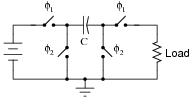Identify the polarity of voltage across the load resistor in the following switched capacitor circuit. Note: the only difference between this circuit and the last is the switching sequence.What difference would it make to the output signal of this operational amplifier circuit if the switching sequence of the switched capacitor network were changed? What difference would it make if the switching frequency were changed?• #### Question 9

Research the resistance equivalence equations for each of these switched-capacitor networks (using N-channel MOSFETs as switches), describing the emulated resistance (R) as a function of switching frequency (f) and capacitance (C):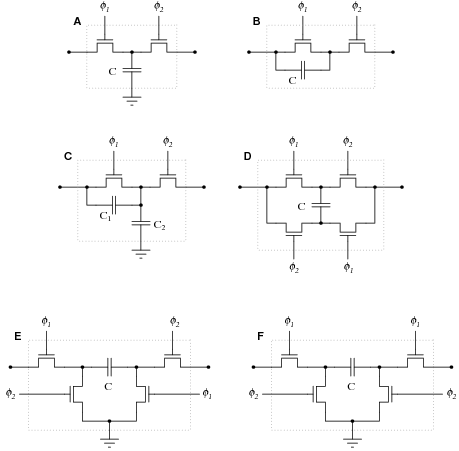Note: φ1 and φ2 are two-phase, non-overlapping clock signals.

• #### Question 10

Given the fact that a switched-capacitor network has the ability to emulate a variable resistance, what advantage(s) can you think of for using switched-capacitor networks inside of analog integrated circuits? Identify some practical applications of this technology.

• #### Question 11

Identify what type of passive filter circuit this is, and then sketch a schematic diagram for an equivalent circuit using a switched-capacitor network instead of a resistor:Also, write an equation describing the cutoff frequency in terms of switching frequency and capacitor values.

• #### Question 12

Identify what type of passive filter circuit this is, and then sketch a schematic diagram for an equivalent circuit using a switched-capacitor network instead of a resistor:Also, write an equation describing the cutoff frequency in terms of switching frequency and capacitor values.

• #### Question 13

A 4.7 μF capacitor (Ci) is charged to a voltage of 4 volts, then connected to the inverting input of an operational amplifier (with a 10 μF feedback capacitor, Cf). What happens to the voltages across Ci and Cf? Explain your answer in detail.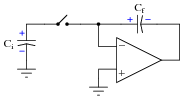• #### Question 14

Many switched capacitor circuits require non-overlapping, two-phase clock signals. Shown here is a schematic diagram of a gate circuit that converts a single clock signal into two complementary, non-overlapping clock signals: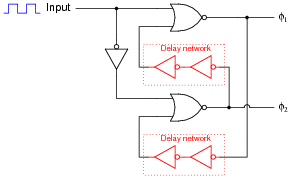Assume that the only gates possessing propagation delays are the double-NOT gates (inside the dotted boxes), whose sole purpose it is to provide a short time delay in the feedback signals. Draw a timing diagram showing the two-phase clock signals (φ1 and φ2) in relation to the input clock waveform, and be prepared to explain how and why this circuit works: